# Microsoft SQL Server R Services - sp_execute_external_script - III

Posted by nielsb on Wednesday, March 21, 2018

This post is the 24:th post about Microsoft SQL Server R Services, and the third that covers `sp_execute_external_script`. I have thought many times that this series has reached its end, and I have many times realised I was wrong. Well, now I can say that this is the last post in the series! I started over a year ago (March 4 2017) with an idea about “wouldn’t it be cool if I wrote a couple of posts about Microsoft SQL Server R Services”! Over one year and 24 posts later, here we are!

This last post talks about `sp_execute_external_script` and a parameter named `@parallel`. When we discuss this parameter, we also touch (a little bit) upon performance aspects of `sp_execute_external_script`.

NOTE: This post has similarities with the Microsoft SQL Server R Services - Internals V post, where I also discussed parallel execution. In that post however I concentrated more on the internal workings , whereas I in this post will not go that deep. In this post I also cover a couple of more things than just the `@parallel` parameter.

## Recap

In the two last posts in this series we discussed `sp_execute_external_script` and the parameters the procedure expects/takes:

 `````` 1 2 3 4 5 6 7 8 9 10 11 `````` ``````sp_execute_external_script @language = N'language', @script = N'script', [ @input_data_1 = ] 'input_data_1' [ , @input_data_1_name = ] N'input_data_1_name' ] [ , @output_data_1_name = N'output_data_1_name' ] [ , @parallel = 0 | 1 ] [ , @params = ] N'@parameter_name data_type [ OUT | OUTPUT ] [ ,...n ]' [ , @parameter1 = ] 'value1' [ OUT | OUTPUT ] [ ,...n ] [ WITH ] [;] ``````

Code Snippet 1: Full Signature of sp_execute_external_script

In Code Snippet 1 we see all parameters for `sp_execute_external_script`, and here is what we have covered so far:

#### Microsoft SQL Server R Services: sp_execute_external_script - I

In sp_execute_external_script - I we discussed:

• `@language` - tells the launchpad service what external engine to use. At the moment R and Python are supported. The parameter is required.
• `@script` - defines the script which the external engine executes. The script can be loaded as a literal value, a parameter or through the R `source(file_name)` command. The parameter is required.
• `@input_data_1` - specifies the input data used by the external script in the form of a Transact-SQL query (`SELECT` only, no procedure calls). The query can only generate one dataset. If more datasets are required, the script must retrieve them from within the script. The parameter is optional.
• `@input_data_1_name` - specifies the name of the variable used to represent the query defined by @input_data_1. The parameter is optional, and defaults to `InputDataset`.
• `@output_data_1_name` - specifies the name of the variable in the external script that contains the data to be returned to SQL Server when the stored procedure completes. The parameter is optional, and defaults to `OutputDataSet`.

All of the above parameter values have to be of the `NVARCHAR` data type.

#### Microsoft SQL Server R Services - sp_execute_external_script - II

The next post sp_execute_external_script - II covered `@params` and `@parameter1`:

• The `@params` parameter is used to define what parameters we use in the execution. The query in `@input_data_1` can use those parameters as well as the external script.
• The parameters defined in the `@params` list need to be added as named parameters to the stored procedure.
• In the case of parameters for the external script; the script references the parameters by name but without the `@` sign
• SQL Server sends the parameters and their values to the SqlSatellite in the packet containing the external script.
• In the “script” packet, preceding the values and the parameter names are hex values indicating the size of the parameter value (data type) and the parameter name.

So, now there is one parameter which we have not talked about: `@parallel`, and - as I mentioned above - that is the topic of this post.

## Housekeeping

Before we “dive” into today’s topics let us look at the code and the tools we use today. This section is here for those who want to follow along in what we are doing in the post.

#### Helper Tools

To help us figure out the things we want, we use Process Monitor, and Process Explorer:

• Process Monitor, is used it to filter TCP traffic. If you want to refresh your memory about how to do it, we covered that in Internals - X as well as in Internals - XIV.
• Process Explorer is used to look at running processes.

#### Code

In this post, we do not use the same database and database objects as we have in last few posts, but something from quite a while back, for example Microsoft SQL Server R Services - Internals V:

 `````` 1 2 3 4 5 6 7 8 9 10 11 12 13 14 15 16 17 18 19 20 21 22 23 24 25 26 27 28 29 30 31 32 33 34 35 36 37 38 39 40 41 42 43 44 45 46 47 48 49 50 51 52 53 54 55 56 57 58 59 60 61 62 63 64 65 66 67 68 69 70 `````` ``````USE master; GO SET NOCOUNT ON; GO DROP DATABASE IF EXISTS TestParallel; GO CREATE DATABASE TestParallel; GO USE TestParallel; GO DROP TABLE IF EXISTS dbo.tb_Rand_3M CREATE TABLE dbo.tb_Rand_3M ( RowID bigint identity PRIMARY KEY, y int NOT NULL, rand1 int NOT NULL, rand2 int NOT NULL, rand3 int NOT NULL, rand4 int NOT NULL, rand5 int NOT NULL, ); INSERT INTO dbo.tb_Rand_3M(y, rand1, rand2, rand3, rand4, rand5) SELECT TOP(3000000) CAST(ABS(CHECKSUM(NEWID())) % 14 AS INT) , CAST(ABS(CHECKSUM(NEWID())) % 20 AS INT) , CAST(ABS(CHECKSUM(NEWID())) % 25 AS INT) , CAST(ABS(CHECKSUM(NEWID())) % 14 AS INT) , CAST(ABS(CHECKSUM(NEWID())) % 50 AS INT) , CAST(ABS(CHECKSUM(NEWID())) % 100 AS INT) FROM sys.objects o1 CROSS JOIN sys.objects o2 CROSS JOIN sys.objects o3 CROSS JOIN sys.objects o4; GO DROP TABLE IF EXISTS dbo.tb_Model; CREATE TABLE dbo.tb_Model ( RowID bigint identity, ModelName nvarchar(50), ModelBin varbinary(max) CONSTRAINT [pk_Model] PRIMARY KEY (RowID) ) GO DROP TABLE IF EXISTS dbo.tb_Rand_30M GO CREATE TABLE dbo.tb_Rand_30M ( RowID bigint identity PRIMARY KEY, y int NOT NULL, rand1 int NOT NULL, rand2 int NOT NULL, rand3 int NOT NULL, rand4 int NOT NULL, rand5 int NOT NULL, ); GO INSERT INTO dbo.tb_Rand_30M(y, rand1, rand2, rand3, rand4, rand5) SELECT TOP(30000000) CAST(ABS(CHECKSUM(NEWID())) % 14 AS INT) , CAST(ABS(CHECKSUM(NEWID())) % 20 AS INT) , CAST(ABS(CHECKSUM(NEWID())) % 25 AS INT) , CAST(ABS(CHECKSUM(NEWID())) % 14 AS INT) , CAST(ABS(CHECKSUM(NEWID())) % 50 AS INT) , CAST(ABS(CHECKSUM(NEWID())) % 100 AS INT) FROM sys.objects o1 CROSS JOIN sys.objects o2 CROSS JOIN sys.objects o3 CROSS JOIN sys.objects o4; GO ``````

Code Snippet 2: Setup of Database, Table and Data

The code above (Code Snippet 2), creates three tables:

• `dbo.tb_Rand_3M` - This table contains the data we want to analyse. Yes, I know - the data is completely useless, but it is a lot of it, and it helps to illustrate what we want to do.
• `dbo.tb_Model` - The purpose of this table is to hold the data science models we create. When we subsequently make predictions against the data we load a model from the table.
• `dbo.tb_Rand_30M` - Similar table to `dbo.tb_Rand_3M` but holds 30 million rows instead of 3 million.

In Code Snippet 2 we also see how we load 3 million records into the `dbo.tb_Rand_3M` as well as 30 million into the `dbo.tb_Rand_30M` tables. Be aware that when you run the code in Code Snippet 2 it may take some time to finish.

The last thing we need to do before we get started in earnest is to create some models which we later use when we talk about the topics for this post. What we want to do with is to create linear regression models, where - ultimately - we want to predict the value of `y` in `dbo.tb_Rand_3M`. We believe `y` is related to `rand1`, `rand2`, `rand3`, `rand4` and `rand5` and that is the bases for the prediction. In reality, there is no relation as such, but in this case, it does not matter - we just want to “play” with some data. We create two models, one using CRANR and one using RevoScaleR functionality, which we then persist to the `dbo.tb_Model` table above:

 `````` 1 2 3 4 5 6 7 8 9 10 11 12 13 14 15 16 17 18 19 20 21 22 23 24 25 26 27 28 29 30 31 32 33 34 35 36 37 38 39 40 41 42 43 `````` ``````-- this creates the CRANR model, using GLM DECLARE @mod varbinary(max); EXEC sp_execute_external_script @language = N'R' , @script = N' myModel <- glm(y ~ rand1 + rand2 + rand3 + rand4 + rand5, data=InputDataSet) model <- serialize(myModel, NULL);' , @input_data_1 = N' SELECT y, rand1, rand2, rand3, rand4, rand5 FROM dbo.tb_Rand_3M TABLESAMPLE(75 PERCENT) REPEATABLE(98074)', @params = N'@model varbinary(max) OUT', @model = @mod OUT INSERT INTO dbo.tb_Model(ModelName, ModelBin) VALUES ('GLM_75Pct', @mod); GO -- this creates the RevoScaleR model DECLARE @mod varbinary(max); EXEC sp_execute_external_script @language = N'R' , @script = N' myModel <- rxLinMod(y ~ rand1 + rand2 + rand3 + rand4 + rand5, data=InputDataSet) model <- serialize(myModel, NULL);' , @input_data_1 = N' SELECT y, rand1, rand2, rand3, rand4, rand5 FROM dbo.tb_Rand_3M TABLESAMPLE(75 PERCENT) REPEATABLE(98074)', @params = N'@model varbinary(max) OUT', @model = @mod OUT; INSERT INTO dbo.tb_Model(ModelName, ModelBin) VALUES ('RxLM_75Pct', @mod); GO ``````

Code Snippet 3: “Creation of CRANR and RevoScaleR Models”

As I said above, the code in Code Snippet 3 creates two models, one using “traditional” `glm`, and one `rxLinMod` from RevoScaleR. We persist the two models to the `dbo.tb_Model` table with a name, so we can retrieve them when we want to run predictions.

Now then, let us start. However, before we look into parallel processing and the `@parallel` parameter and all the other “cool stuff” let us recap what we have talked about in previous posts.

## Background

In quite a few posts we have discussed how the launchpad service creates multiple RTerm processes when we execute `sp_execute_external_script`, and by default one of those processes executes the work. We can see the behaviour if we execute following code:

 `````` 1 2 3 4 5 6 7 8 9 10 11 12 13 14 15 16 17 18 19 20 21 22 23 24 25 `````` `````` DECLARE @model varbinary(max) = (SELECT TOP(1) ModelBin FROM dbo.tb_Model WHERE ModelName = 'RxLM_75Pct'); EXEC sp_execute_external_script @language = N'R', @script = N' Sys.sleep(10) mod <- unserialize(model); cat(paste0("R Process ID = ", Sys.getpid())) cat("\n") cat(paste0("Nmbr rows = ", nrow(InputDataSet))) cat("\n") cat("----------------") cat("\n") OutputDataSet <- data.frame(rxPredict(modelObject = mod, data = InputDataSet, outData = NULL, type = "response", writeModelVars = FALSE, overwrite = TRUE));', @input_data_1 = N'SELECT TOP(2800000) y, rand1, rand2, rand3, rand4, rand5 FROM dbo.tb_Rand_3M WHERE rand5 >= 10', @params = N'@model varbinary(max)', @model = @model; ``````

**Code Snippet 4:Executing Prediction

In Code Snippet 4 we see how we, to begin with, retrieve the binary representation of the persisted model and use it as a parameter passed into the script. In the script, we first deserialise the binary model passed in, and from there we use it to predict `y`, based on the input data. The data passed in is the dataset we `SELECT` in `@input_data_1`, and the prediction function we use is the RevoScaleR `rxPredict` function. Finally, the predicted values come back in the `OutputDataSet`. Oh, the `Sys.sleep` at the first line of the script is so we, when we use Process Explorer, more easily can see what is happening. The part of the script with the `cat` statements is also to make what we see clearer.

NOTE: In future posts, outside of this series, the plan is to talk more about RevoScaleR functions.

When we execute the code, a resultset comes back and a print of the `cat` statements as below: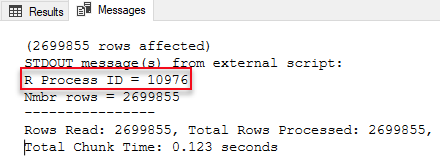Figure 1: Output of cat

We see in Figure 1 the process id of the RTerm the script ran in, and the number of rows that the external engine processed. Now let us check with Process Explorer what happens when we execute the same code:

• Run Process Explorer as admin.
• Restart the launchpad service (this is to clean-up any RTerm processes).
• Navigate to the `Launchpad.exe` process in Process Explorer.
• Execute the code in Code Snippet 4.

While the code is executing, look under the `Launchpad.exe` process in Process Explorer and you see something like so: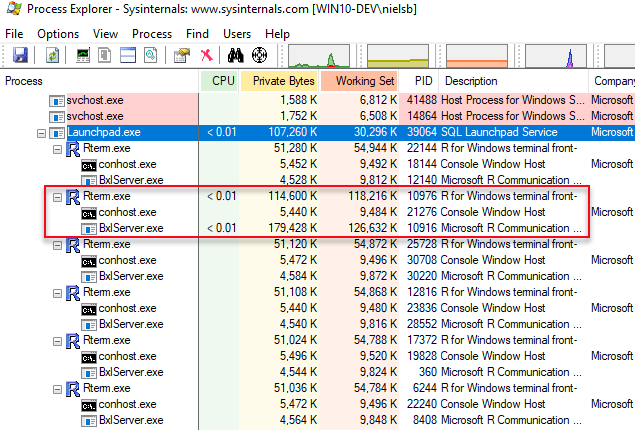Figure 2: Process Explorer Output

We see in Figure 2 multiple RTerm processes, hosting BxlServer processes and this matches pretty good with what we discussed in previous posts about the launchpad service, RTerm and BxlServer processes and so forth, and we try to illustrate that like this: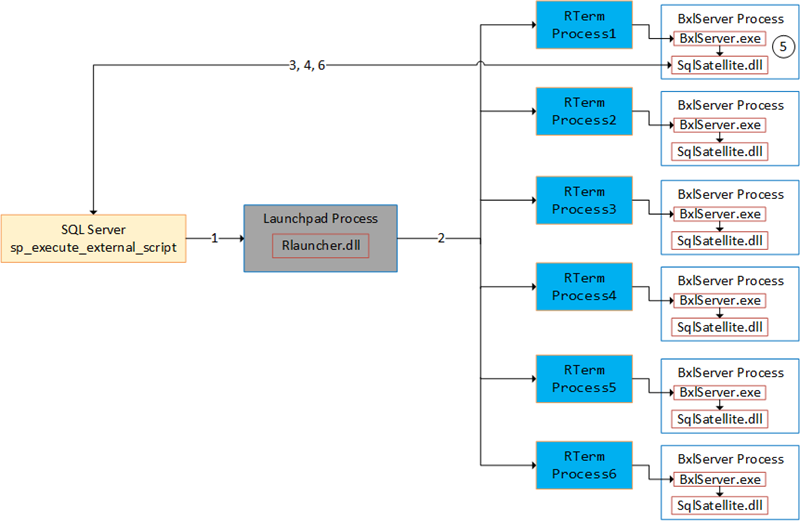Figure 3: Process Flow

In Figure 3 we see what happens when we execute `sp_execute_external_script` and the numbers in the figure stands for:

1. We call `sp_execute_external_script` and SQL Server calls into the launchpad service.
2. The launchpad service creates RTerm processes which in turn creates BxlServer processes. One process becomes the executing process (outlined in red in Figure 2).
3. A TCP connection from the SqlSatellite in the executing process gets established.
4. SQL server sends input data to the SqlSatellite.
5. The `BxlServer.exe` does the processing.
6. SQL Server receives data back from the SqlSatellite.

## Parallel Processing

Let us now look at parallel execution. When you read documentation about SQL Server R Services it always mentions how efficient it is to execute external scripts as one can utilise SQL’s parallelism. How does this work then? In “normal” SQL Server parallelism, SQL Server decides whether to execute a statement in parallel or not, and the `MAXDOP` setting defines the number of processors executing the statement.

#### `@parallel`

In SQL Server R Services it works in a similar way, except that you have to say that you want to execute in parallel explicitly. To indicate you want parallel processing, you use the `@parallel` parameter, as so: `@parallel = 1`. The `@parallel` parameter is optional and by default set to 0 (no parallelism). This code shows it in action:

 `````` 1 2 3 4 5 6 7 8 9 10 11 12 13 14 15 16 17 18 19 20 21 22 23 24 25 26 27 28 `````` ``````DECLARE @model varbinary(max) = (SELECT TOP(1) ModelBin FROM dbo.tb_Model WHERE ModelName = 'RxLM_75Pct'); EXEC sp_execute_external_script @language = N'R', @script = N' Sys.sleep(10) mod <- unserialize(model); cat(paste0("R Process ID = ", Sys.getpid())) cat("\n") cat(paste0("Nmbr rows = ", nrow(InputDataSet))) cat("\n") cat("----------------") cat("\n") OutputDataSet <- data.frame(rxPredict(modelObject = mod, data = InputDataSet, outData = NULL, type = "response", writeModelVars = FALSE, overwrite = TRUE));', @input_data_1 = N'SELECT TOP(2800000) y, rand1, rand2, rand3, rand4, rand5 FROM dbo.tb_Rand_3M WHERE rand5 >= 10 OPTION(querytraceon 8649, MAXDOP 4)', @parallel = 1, @params = N'@model varbinary(max)', @model = @model WITH RESULT SETS ((Y_predict float)); GO ``````

Code Snippet 5: Parallel Execution External Script

As you see, Code Snippet 5 looks more or less like Code Snippet 4, the only difference being:

• `OPTION(querytraceon 8649, MAXDOP 4)` at the end of the input query.
• `@parallel = 1`.
• `WITH RESULT SETS ((Y_predict float))`.

The `@parallel = 1` probably does not need any explanation - we want to run in parallel, but the `OPTION(querytraceon 8649, MAXDOP 4)` may need some clarification. When I played with researched the code for this post I had problems with getting a parallel query plan, so I try to force parallelism by setting the query trace flag 8649 explicitly. When using `@parallel = 1` you must specify a `WITH RESULT SETS` clause with output schema, regardless if you actually return a resultset or not.

So let us now see what it looks like when we execute the code in Code Snippet 5. For this we use Process Explorer in the same way as we used it above:

• Run Process Explorer as admin.
• Restart the launchpad service (this is to clean-up any RTerm processes).
• Navigate to the `Launchpad.exe` process in Process Explorer.

Execute the code in Code Snippet 5, and while it executes look under the `Launchpad.exe` and see if it is different from above: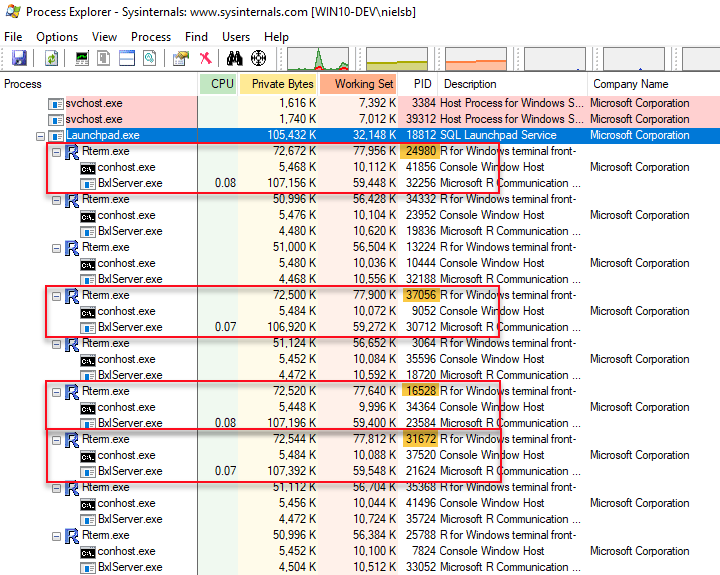Figure 4: Parallel Execution

Yes, what we see in Figure 4 is different than Figure 2, and we see how we now have four RTerm processes active (outlined in red). When we look at the output of the `cat` statements (below) we see that we have four different R process id’s, and they match the highlighted (in yellow) process id’s of the active RTerm processes in Figure 4: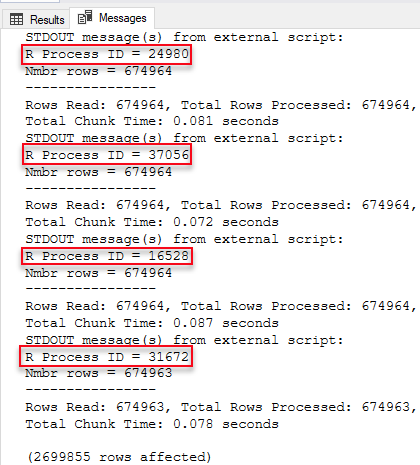Figure 5: SSMS Output

So Figure 5 shows that we ran the `rxPredict` function in parallel using 4 processes, and the reason for 4 processes was the `MAXDOP` setting (4). So how does this work then, is there a coordinator somewhere distributing the work, or what? Let us try and figure that out, and to help us we use Process Monitor (for now we can exit Process Explorer).

Run Process Monitor as admin and set a filter where we see connections to the `BxlServer.exe` process. To set the filter; under the Filter menu in Process Monitor click the Filter menu item to see the “Process Monitor Filter” dialog. To create the filter we enter the conditions we want to match:

• The Process Name (from the first drop-down) should be is (from the second drop down): `BxlServer.exe`.
• Operation (first drop-down) is (second drop-down): “TCP Connect”.
• Click OK “Process Monitor Filter” dialog.

The filter we created filters out anything but “TCP Connect” events against the `BxlServer.exe` process. We are interested in the “TCP Connect” events because when no parallelism is in play, there is only one connection between SQL Server and the BxlServer process - or rather the SqlSatellite which BxlServer loads into its process.

To see what happens, execute the code in Code Snippet 5 and watch the output from Process Monitor: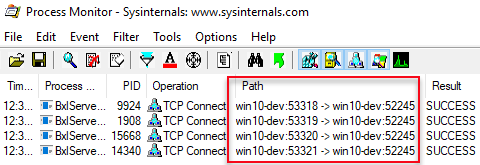Figure 6: Multiple Connections

Notice, in Figure 6, how there are four connections, and when we look in the `Path` column we see how the connections are all made to port 52245 from ports: 53318, 53319, 53320 and 53321. Port 52245 is SQL Server, and the other ports are SqlSatellite. OK, so four connections are made up front, what about sending data to the SqlSatellite? Change the filter, instead of “TCP Connect”, to filter on “TCP Receive”, e.g. what the SqlSatellite(s) receives. Execute the code in Code Snippet 5 again and see what Process Monitor outputs:Figure 7: Multiple Setup Packets

Wow, that was many events! In Figure 7 we see the 12 first packets and how they sit in 4 groups for 4 distinct port numbers. The packets we see are the packets we discussed in quite a few posts, Internals X, XI and XII amongst them, and the packets are the authentication packets SQL Server sends to the SqlSatellite and the packet containing the external script. So it looks like 4 SqlSatellites receives the same setup and script packets. When we scroll further down in the output we see how SQL Server sends input-data packets to the SqlSatellites:Figure 8: Input Data Packets

Figure 8 shows data packets sent from SQL Server (port 52245) to SqlSatellites and ports 55124 and 55126. If we browse through the whole output, we can see how SQL Server sends data to all four ports we see in Figure 7. When SQL Server sends the input-data packets, each SqlSatellite receives different data.

For data that comes back to SQL Server, the flow is the same; each SqlSatellite sends back “its” own data, and the figure below illustrates the full flow: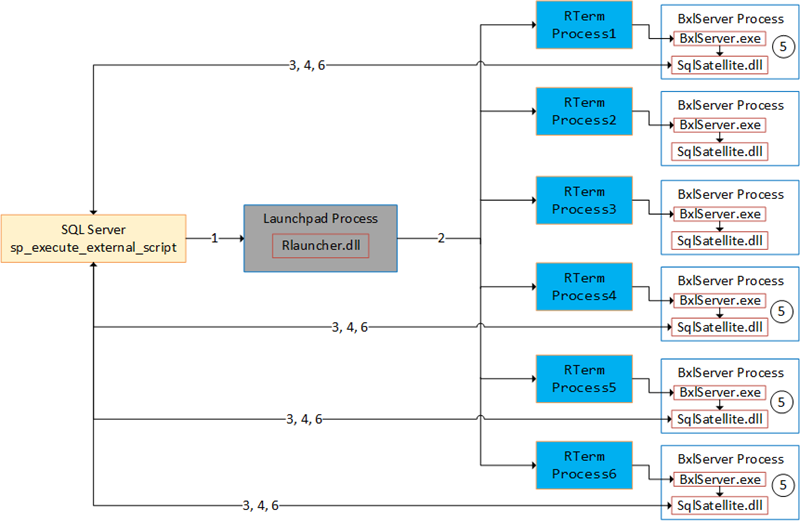Figure 9: Complete Flow

Figure 9 tries to illustrate executing external script in parallel, and the numbers in the figure are the same as for Figure 3:

1. We call `sp_execute_external_script` and SQL Server calls into the launchpad service.
2. The launchpad service creates RTerm processes which in turn creates BxlServer processes. Based on `MAXDOP`, multiple executing processes “spin up”.
3. TCP connections from the SqlSatellite in the executing processes get established.
4. SQL server sends input data to the SqlSatellites.
5. The `BxlServer.exe` processes the data.
6. SQL Server receives data back from the individual SqlSatellite.

So to answer the question about if there is a coordinator somewhere; SQL Server is the coordinator, and SQL Server sends data to the various SqlSatellites and the hosting BxlServer processes. It is important to note how the SqlSatellite and BxlServer processes, processes the data independently, which means that you do not want to do parallel execution through `@parallel = 1` if your data has dependencies between different rows, like creating models. Later in this post we cover how you can execute in parallel even if you have dependencies between rows.

A final word about parallel execution via `@parallel`: the requirement for parallel execution is that SQL Server can create a parallel execution plan of the input query. If SQL Server can do that, you get parallel execution. This also means that you can use CRANR functions instead of RevoScaleR functions, so in Code Snippet 5 we can replace `rxPredict` with CRANR `predict`.

#### `@r_rowsPerRead`

Sometimes you are in a situation where you need to process more data than fits into memory, and this can especially be true when you use CRANR functions which are not optimised to handle large datasets. That is where streaming of data comes in handy and the `@r_rowsPerRead` parameter. The `@r_rowsPerRead` parameter is not part of the signature of `sp_execute_external_script`, but it is still a valid parameter. It allows you to control the number of rows passed during streaming, by specifying an integer value for the parameter and adding the parameter to the `@params` collection:

 `````` 1 2 3 4 5 6 7 8 9 10 11 12 13 14 15 16 17 18 19 20 21 22 23 24 `````` ``````DECLARE @model varbinary(max) = (SELECT TOP(1) ModelBin FROM dbo.tb_Model WHERE ModelName = 'GLM_75Pct'); EXEC sp_execute_external_script @language = N'R', @script = N' mod <- unserialize(model); cat(paste0("R Process ID = ", Sys.getpid())) cat("\n") cat(paste0("Nmbr rows = ", nrow(InputDataSet))) cat("\n") cat("----------------") cat("\n") OutputDataSet <- data.frame(predict(mod, newdata = InputDataSet, type = "response"))', @input_data_1 = N' SELECT TOP(1000) y, rand1, rand2, rand3, rand4, rand5 FROM dbo.tb_Rand_3M', @params = N'@model varbinary(max), @r_rowsPerRead int', @model = @model, @r_rowsPerRead = 250 WITH RESULT SETS ((Y_predict float)); ``````

Code Snippet 6: CRANR Rows Per Read

The code in Code Snippet 6 is similar to Code Snippet 5, however in Code Snippet 6 we use the CRANR `predict` function, and we also stream the data by defining the `@r_rowsPerRead` parameter and setting it to 250. The output after we execute the code looks like so: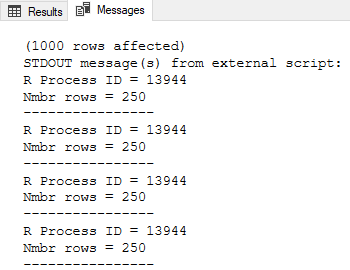Figure 10: Streaming Data

The output from SSMS, which we see in Figure 10, shows how 250 rows have been processed four times by the same process id. What about streaming and parallel processing? Sure, that works as well, but I do not cover it in this post. OK, so how does the streaming work then, does SQL Server send batches of data to the SqlSatellite(s) as we saw when we executed in parallel? Let us try to find out, and we start with changing the code a little bit to make it simpler:

 `````` 1 2 3 4 5 6 7 8 9 10 11 12 13 14 `````` ``````EXEC sp_execute_external_script @language = N'R', @script = N' cat(paste0("R Process ID = ", Sys.getpid())) cat("\n") cat(paste0("Nmbr rows = ", nrow(InputDataSet))) cat("\n") cat("----------------") cat("\n") OutputDataSet <- InputDataSet', @input_data_1 = N' SELECT TOP(100) y, rand1, rand2, rand3, rand4, rand5 FROM dbo.tb_Rand_3M'; ``````

Code Snippet 7: Simplified Code - No Streaming

As you see in Code Snippet 7 we no longer use a model, and we have also “scrapped” `@r_rowsPerRead`. In essence, the code looks like most of the code used throughout this series. When we use Process Monitor with the same filter as above, the output looks like this when we execute the code in Code Snippet 7: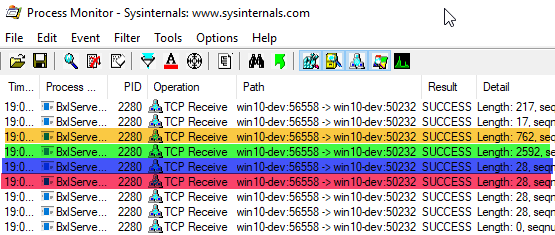Figure 11: Simple Output

Look at the highlighted rows in Figure 11:

• Yellow, length of 762 - this is the packet with the script.
• Green, length of 2592 - the input data (the 100 rows).
• Blue, length of 28 - end of the script.
• Red, length of 28 - end of the resultset.

Compare what you see above with the output for code like so:

 `````` 1 2 3 4 5 6 7 8 9 10 11 12 13 14 15 16 `````` ``````EXEC sp_execute_external_script @language = N'R', @script = N' Sys.sleep(10) cat(paste0("R Process ID = ", Sys.getpid())) cat("\n") cat(paste0("Nmbr rows = ", nrow(InputDataSet))) cat("\n") cat("----------------") cat("\n") OutputDataSet <- InputDataSet', @input_data_1 = N' SELECT TOP(100) y, rand1, rand2, rand3, rand4, rand5 FROM dbo.tb_Rand_3M', @params = N'@r_rowsPerRead int', @r_rowsPerRead = 25; ``````

Code Snippet 8: Simplified Code - No Streaming

In Code Snippet 8 we add the `@r_rowsPerRead` parameter, and we set it to 25: stream 25 rows at a time. You see how we also have a `Sys.sleep(10)` in the script. That is to make what happens more transparent. Execute the code and watch the output from Process Monitor: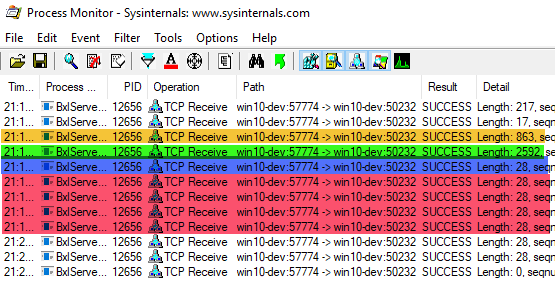Figure 12: Streaming Output

Oh, that is interesting! In Figure 12 we now see how the three first highlighted packets are the same as in Figure 11, highlighted in yellow, green and blue. The only slight difference is the script packet, where the packet in Figure 12 is somewhat bigger. The reason for the packet being bigger in Figure 12 is that the `@r_rowsPerRead` parameter is part of the script packet. What we see makes it clear that SQL Server sends the whole resultset packet (green) to the SqlSatellite in one go, and the streaming is not because of batching from SQL Server. The streaming is due to SqlSatellite receiving an end of resultset packet (red), and when it receives the packet, it streams in the number of rows as per the `@r_rowsPerRead` parameter.

## SQL Server Compute Context and Parallel Processing

UPDATE: Originally I covered the SQL Server Compute Context in this post. However, it turns out that; how I thought things worked was not necessarily true, so I wrote the post sp_execute_external_script and SQL Compute Context - I, to try and correct my mistakes. So if you are interested in how the SQL Server compute context works from `sp_execute_external_script` go over and have a look.

## Summary

In this, the last post in the [Microsoft SQL Server R Services series we discussed:

• Parallel processing via the `@parallel` parameter.
• Streaming of data using `@r_rowsPerRead.`
• SQL Server compute-context.

#### `@parallel`

• By setting the `@parallel` parameter to 1, you can get parallel processing if the query SQL Server sends to the SqlSatellite can be parallelised.
• The parallelism you get is “trivial” parallelism; each task processes and returns the data individually.
• Useful if there are no dependencies between rows.
• Can be used with CRANR functions.

#### `@r_rowsPerRead`

• `@r_rowsPerRead` allow you to stream data from SQL Server to the SqlSatellite(s).
• All data is pushed from SQL Server in one go, but the SqlSatellite(s) reads the data per the value of the parameter.
• `@r_rowsPerRead` can be used in conjunction with `@parallel`.

#### Compute Context

The post sp_execute_external_script and SQL Compute Context - I covers SQL Server Compute Context.

## ~ Finally

If you have comments, questions etc., please comment on this post or ping me. As mentioned, this is the last post in this series: I have had a lot of fun writing this and I have learned a lot. Hope you - my readers - have enjoyed it as well!